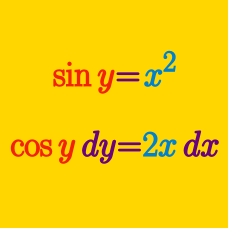Calculus

# Implicit Differentiation - Trigonometric Functions

If $10xy=\cot(xy),$ what is $\displaystyle \frac{dy}{dx}?$

If $7\cos x \sin y=11,$ what is $\displaystyle \frac{dy}{dx}?$

If $\sin (x+3y)=y^{4}\cos x,$ what is $\frac{dy}{dx}?$

Let $y = \sin(6x + 20y)$. The slope of the tangent at the point $(x,y) = (0,0)$ can be expressed as $-\frac{a}{b}$, where $a$ and $b$ are coprime positive integers. What is the value of $a+b$?

If $x^2y^2+x\sin y=13,$ what is $\displaystyle \frac{dy}{dx}?$

×